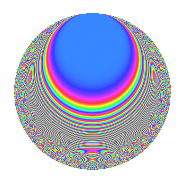# Properties

 Label 1155.2.l.eLevel 1155 Weight 2 Character orbit 1155.l Analytic conductor 9.223 Analytic rank 0 Dimension 40 CM No

# Learn more about

## Newspace parameters

 Level: $$N$$ = $$1155 = 3 \cdot 5 \cdot 7 \cdot 11$$ Weight: $$k$$ = $$2$$ Character orbit: $$[\chi]$$ = 1155.l (of order $$2$$ and degree $$1$$)

## Newform invariants

 Self dual: No Analytic conductor: $$9.22272143346$$ Analytic rank: $$0$$ Dimension: $$40$$ Sato-Tate group: $\mathrm{SU}(2)[C_{2}]$

## $q$-expansion

The dimension is sufficiently large that we do not compute an algebraic $$q$$-expansion, but we have computed the trace expansion.

 $$\operatorname{Tr}(f)(q) =$$ $$40q$$ $$\mathstrut -\mathstrut 4q^{2}$$ $$\mathstrut -\mathstrut 4q^{3}$$ $$\mathstrut +\mathstrut 44q^{4}$$ $$\mathstrut -\mathstrut 4q^{6}$$ $$\mathstrut -\mathstrut 12q^{8}$$ $$\mathstrut -\mathstrut 2q^{9}$$ $$\mathstrut +\mathstrut O(q^{10})$$ $$\operatorname{Tr}(f)(q) =$$ $$40q$$ $$\mathstrut -\mathstrut 4q^{2}$$ $$\mathstrut -\mathstrut 4q^{3}$$ $$\mathstrut +\mathstrut 44q^{4}$$ $$\mathstrut -\mathstrut 4q^{6}$$ $$\mathstrut -\mathstrut 12q^{8}$$ $$\mathstrut -\mathstrut 2q^{9}$$ $$\mathstrut -\mathstrut 6q^{11}$$ $$\mathstrut +\mathstrut 8q^{12}$$ $$\mathstrut +\mathstrut 2q^{15}$$ $$\mathstrut +\mathstrut 68q^{16}$$ $$\mathstrut +\mathstrut 40q^{18}$$ $$\mathstrut -\mathstrut 2q^{21}$$ $$\mathstrut -\mathstrut 4q^{22}$$ $$\mathstrut -\mathstrut 12q^{24}$$ $$\mathstrut -\mathstrut 40q^{25}$$ $$\mathstrut +\mathstrut 32q^{27}$$ $$\mathstrut -\mathstrut 24q^{29}$$ $$\mathstrut -\mathstrut 8q^{31}$$ $$\mathstrut +\mathstrut 52q^{32}$$ $$\mathstrut -\mathstrut 16q^{33}$$ $$\mathstrut +\mathstrut 32q^{34}$$ $$\mathstrut +\mathstrut 40q^{35}$$ $$\mathstrut -\mathstrut 48q^{37}$$ $$\mathstrut +\mathstrut 66q^{39}$$ $$\mathstrut -\mathstrut 16q^{41}$$ $$\mathstrut +\mathstrut 32q^{44}$$ $$\mathstrut +\mathstrut 32q^{48}$$ $$\mathstrut -\mathstrut 40q^{49}$$ $$\mathstrut +\mathstrut 4q^{50}$$ $$\mathstrut -\mathstrut 14q^{51}$$ $$\mathstrut -\mathstrut 72q^{54}$$ $$\mathstrut +\mathstrut 8q^{55}$$ $$\mathstrut -\mathstrut 24q^{57}$$ $$\mathstrut -\mathstrut 80q^{58}$$ $$\mathstrut +\mathstrut 24q^{60}$$ $$\mathstrut +\mathstrut 48q^{62}$$ $$\mathstrut +\mathstrut 76q^{64}$$ $$\mathstrut +\mathstrut 4q^{65}$$ $$\mathstrut -\mathstrut 76q^{66}$$ $$\mathstrut -\mathstrut 48q^{67}$$ $$\mathstrut -\mathstrut 32q^{68}$$ $$\mathstrut -\mathstrut 20q^{69}$$ $$\mathstrut -\mathstrut 4q^{70}$$ $$\mathstrut +\mathstrut 128q^{72}$$ $$\mathstrut +\mathstrut 4q^{75}$$ $$\mathstrut -\mathstrut 8q^{77}$$ $$\mathstrut -\mathstrut 28q^{78}$$ $$\mathstrut +\mathstrut 50q^{81}$$ $$\mathstrut -\mathstrut 32q^{82}$$ $$\mathstrut -\mathstrut 24q^{83}$$ $$\mathstrut -\mathstrut 24q^{84}$$ $$\mathstrut -\mathstrut 32q^{87}$$ $$\mathstrut -\mathstrut 16q^{88}$$ $$\mathstrut +\mathstrut 8q^{90}$$ $$\mathstrut -\mathstrut 4q^{91}$$ $$\mathstrut -\mathstrut 20q^{93}$$ $$\mathstrut -\mathstrut 12q^{95}$$ $$\mathstrut +\mathstrut 12q^{96}$$ $$\mathstrut +\mathstrut 100q^{97}$$ $$\mathstrut +\mathstrut 4q^{98}$$ $$\mathstrut -\mathstrut 54q^{99}$$ $$\mathstrut +\mathstrut O(q^{100})$$

## Embeddings

For each embedding $$\iota_m$$ of the coefficient field, the values $$\iota_m(a_n)$$ are shown below.

For more information on an embedded modular form you can click on its label.

Label $$a_{2}$$ $$a_{3}$$ $$a_{4}$$ $$a_{5}$$ $$a_{6}$$ $$a_{7}$$ $$a_{8}$$ $$a_{9}$$ $$a_{10}$$
1121.1 −2.79309 0.351991 1.69591i 5.80135 1.00000i −0.983141 + 4.73682i 1.00000i −10.6175 −2.75221 1.19389i 2.79309i
1121.2 −2.79309 0.351991 + 1.69591i 5.80135 1.00000i −0.983141 4.73682i 1.00000i −10.6175 −2.75221 + 1.19389i 2.79309i
1121.3 −2.55898 −0.371837 1.69167i 4.54836 1.00000i 0.951521 + 4.32893i 1.00000i −6.52118 −2.72347 + 1.25805i 2.55898i
1121.4 −2.55898 −0.371837 + 1.69167i 4.54836 1.00000i 0.951521 4.32893i 1.00000i −6.52118 −2.72347 1.25805i 2.55898i
1121.5 −2.21445 1.64596 0.539264i 2.90381 1.00000i −3.64491 + 1.19418i 1.00000i −2.00144 2.41839 1.77522i 2.21445i
1121.6 −2.21445 1.64596 + 0.539264i 2.90381 1.00000i −3.64491 1.19418i 1.00000i −2.00144 2.41839 + 1.77522i 2.21445i
1121.7 −2.04560 −1.22443 1.22506i 2.18447 1.00000i 2.50470 + 2.50597i 1.00000i −0.377355 −0.00153018 + 3.00000i 2.04560i
1121.8 −2.04560 −1.22443 + 1.22506i 2.18447 1.00000i 2.50470 2.50597i 1.00000i −0.377355 −0.00153018 3.00000i 2.04560i
1121.9 −1.88704 1.57144 0.728399i 1.56093 1.00000i −2.96538 + 1.37452i 1.00000i 0.828540 1.93887 2.28928i 1.88704i
1121.10 −1.88704 1.57144 + 0.728399i 1.56093 1.00000i −2.96538 1.37452i 1.00000i 0.828540 1.93887 + 2.28928i 1.88704i
1121.11 −1.80850 −1.28663 + 1.15956i 1.27068 1.00000i 2.32688 2.09707i 1.00000i 1.31898 0.310844 2.98385i 1.80850i
1121.12 −1.80850 −1.28663 1.15956i 1.27068 1.00000i 2.32688 + 2.09707i 1.00000i 1.31898 0.310844 + 2.98385i 1.80850i
1121.13 −1.71054 −0.134822 1.72680i 0.925948 1.00000i 0.230619 + 2.95375i 1.00000i 1.83721 −2.96365 + 0.465620i 1.71054i
1121.14 −1.71054 −0.134822 + 1.72680i 0.925948 1.00000i 0.230619 2.95375i 1.00000i 1.83721 −2.96365 0.465620i 1.71054i
1121.15 −0.805231 −0.972634 + 1.43317i −1.35160 1.00000i 0.783195 1.15404i 1.00000i 2.69881 −1.10797 2.78790i 0.805231i
1121.16 −0.805231 −0.972634 1.43317i −1.35160 1.00000i 0.783195 + 1.15404i 1.00000i 2.69881 −1.10797 + 2.78790i 0.805231i
1121.17 −0.542651 −1.58724 + 0.693309i −1.70553 1.00000i 0.861316 0.376225i 1.00000i 2.01081 2.03865 2.20089i 0.542651i
1121.18 −0.542651 −1.58724 0.693309i −1.70553 1.00000i 0.861316 + 0.376225i 1.00000i 2.01081 2.03865 + 2.20089i 0.542651i
1121.19 0.164753 1.34733 1.08845i −1.97286 1.00000i 0.221976 0.179324i 1.00000i −0.654539 0.630571 2.93298i 0.164753i
1121.20 0.164753 1.34733 + 1.08845i −1.97286 1.00000i 0.221976 + 0.179324i 1.00000i −0.654539 0.630571 + 2.93298i 0.164753i
See all 40 embeddings
 $$n$$: e.g. 2-40 or 990-1000 Embeddings: e.g. 1-3 or 1121.40 Significant digits: Format: Complex embeddings Normalized embeddings Satake parameters Satake angles

## Inner twists

This newform does not have CM; other inner twists have not been computed.

## Hecke kernels

This newform can be constructed as the intersection of the kernels of the following linear operators acting on $$S_{2}^{\mathrm{new}}(1155, [\chi])$$:

 $$T_{2}^{20} + \cdots$$ $$T_{17}^{20} - \cdots$$